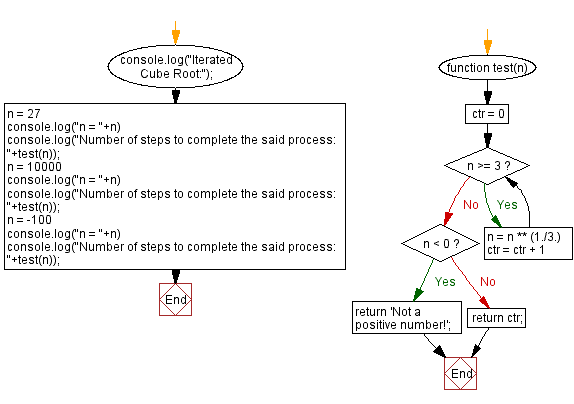# JavaScript: Iterated Cube Root

## JavaScript Math: Exercise-92 with Solution

Write a Python program that takes a positive integer and calculates the cube root of the number until it is less than three. Return the number of steps to complete this process.

Test Data:
(27) -> 2
(10000) -> 2
(-100) -> "Not a positive number!"

Sample Solution:

HTML Code:

``````<!DOCTYPE html>
<html>
<meta charset="utf-8">
<title>JavaScript program to Iterated Cube Root</title>
<body>

</body>
</html>
```
```

JavaScript Code:

``````function test(n)
{
ctr = 0
while(n >= 3)
{
n =  n ** (1./3.)
ctr = ctr + 1
}
if (n < 0)
return 'Not a positive number!';
else
return ctr;
}
console.log("Iterated Cube Root:");
n = 27
console.log("n = "+n)
console.log("Number of steps to complete the said process: "+test(n));
n = 10000
console.log("n = "+n)
console.log("Number of steps to complete the said process: "+test(n));
n = -100
console.log("n = "+n)
console.log("Number of steps to complete the said process: "+test(n));
```
```

Sample Output:

```Iterated Cube Root:
n = 27
Number of steps to complete the said process: 2
n = 10000
Number of steps to complete the said process: 2
n = -100
Number of steps to complete the said process: Not a positive number!
```

Flowchart:Live Demo:

See the Pen javascript-math-exercise-92 by w3resource (@w3resource) on CodePen.

Improve this sample solution and post your code through Disqus

What is the difficulty level of this exercise?

Test your Programming skills with w3resource's quiz.

﻿